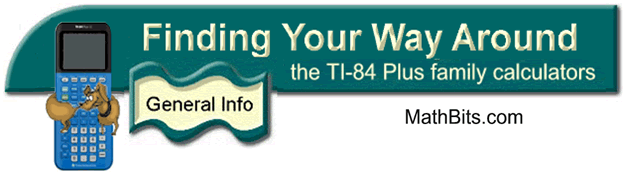Basic Graphing
Both Classic Mode and MathPrint Mode

 1.  Enter equations into "Y =" area.  (Be sure the PLOTS at the top of window are turned off.)  When the = sign is highlighted, the graph will be displayed.                                        Typing Options:                                           Y1 = -0.5x + 3                                          Y1 = -1/2x + 3                                          Y1 = (-1/2)x +3 The symbol to the far left of the equation indicates the style of graph you will see.  For example, the -0 symbol will be an animated "bubble" with a trailing graph.  To change these options, move to the far left and hit ENTER.  With each hit, another choice will appear.  If you go past an option, keep hitting ENTER and it will reappear.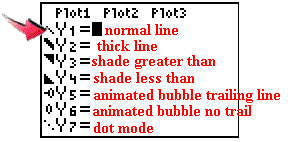If the Plots at the top are highlighted, move to them and hit ENTER to deselect.Since the equal sign in the second graph, y = 2x, is not highlighted, the line will not be graphed.  To highlight the = sign, move to it and press ENTER.2.  Choose the viewing window.  The ZOOM key will let you chose various pre-set windows.  The most common choice is #6 ZStandard which gives a [-10,10] by [-10,10] window. Note: This window may be simply referred to as a 10 x 10 window, implying 10 units in all directions.If you prefer, you can control your own viewing window by choosing WINDOW.  The window shown above was the result of choosing #6 ZStandard.
 3.  Hit GRAPH to see your graphs.  Notice that the second graph, y = 2x, did not graph.  To see the graph, the = sign must be highlighted.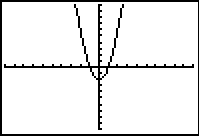4.  If you need to find the roots of the equation (the x-intercepts) you may choose #2 ZERO from the CALCULATE menu under CALC (above the TRACE key).  The calculator will prompt you for responses.  If you need to find points of intersection between two graphs, you may choose #5 INTERSECT from this same menu.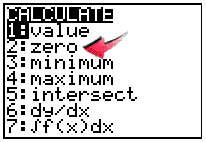5.  If you need to see values relating to the graph, go to the TABLE (2nd GRAPH).  You can control what you see in the table by going to TBLSET (2nd WINDOW).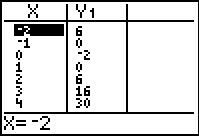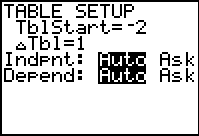Finding Your Way Around TABLE of  CONTENTS